# Partial Differential Equations Paul Heckbert Computer Science Department

• Slides: 13Partial Differential Equations Paul Heckbert Computer Science Department Carnegie Mellon University 7 Nov. 2000 15 -859 B - Introduction to Scientific Computing 1Differential Equation Classes 1 • dimension of unknown: – ordinary differential equation (ODE) – unknown is a function of one variable, e. g. y(t) – partial differential equation (PDE) – unknown is a function of multiple variables, e. g. u(t, x, y) • number of equations: – single differential equation, e. g. y’=y – system of differential equations (coupled), e. g. y 1’=y 2, y 2’=-g • order – nth order DE has nth derivative, and no higher, e. g. y’’=-g 7 Nov. 2000 15 -859 B - Introduction to Scientific Computing 2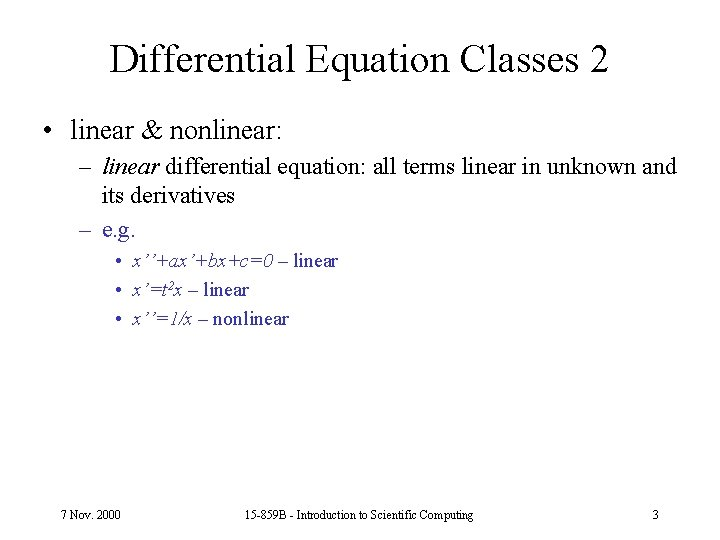Differential Equation Classes 2 • linear & nonlinear: – linear differential equation: all terms linear in unknown and its derivatives – e. g. • x’’+ax’+bx+c=0 – linear • x’=t 2 x – linear • x’’=1/x – nonlinear 7 Nov. 2000 15 -859 B - Introduction to Scientific Computing 3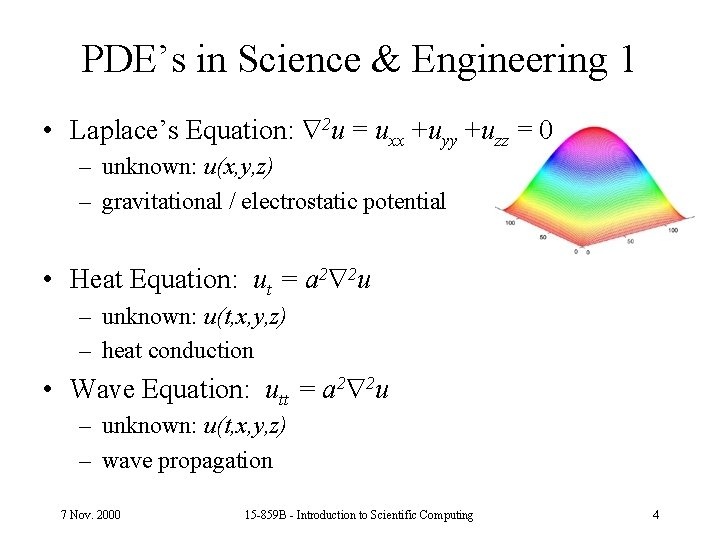PDE’s in Science & Engineering 1 • Laplace’s Equation: 2 u = uxx +uyy +uzz = 0 – unknown: u(x, y, z) – gravitational / electrostatic potential • Heat Equation: ut = a 2 2 u – unknown: u(t, x, y, z) – heat conduction • Wave Equation: utt = a 2 2 u – unknown: u(t, x, y, z) – wave propagation 7 Nov. 2000 15 -859 B - Introduction to Scientific Computing 4PDE’s in Science & Engineering 2 • Schrödinger Wave Equation – quantum mechanics – (electron probability densities) • Navier-Stokes Equation – fluid flow (fluid velocity & pressure) 7 Nov. 2000 15 -859 B - Introduction to Scientific Computing 5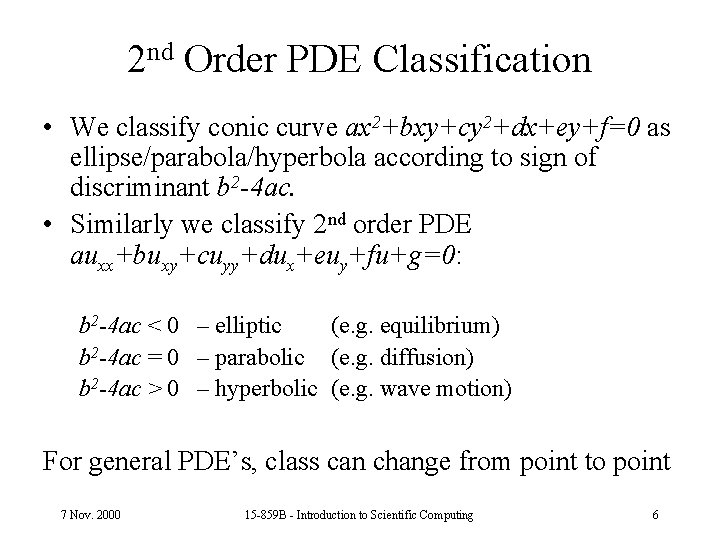2 nd Order PDE Classification • We classify conic curve ax 2+bxy+cy 2+dx+ey+f=0 as ellipse/parabola/hyperbola according to sign of discriminant b 2 -4 ac. • Similarly we classify 2 nd order PDE auxx+buxy+cuyy+dux+euy+fu+g=0: b 2 -4 ac < 0 – elliptic (e. g. equilibrium) b 2 -4 ac = 0 – parabolic (e. g. diffusion) b 2 -4 ac > 0 – hyperbolic (e. g. wave motion) For general PDE’s, class can change from point to point 7 Nov. 2000 15 -859 B - Introduction to Scientific Computing 6Example: Wave Equation • • • utt = c uxx for 0 x 1, t 0 initial cond. : u(0, x)=sin( x)+x+2, ut(0, x)=4 sin(2 x) boundary cond. : u(t, 0) = 2, u(t, 1)=3 c=1 unknown: u(t, x) • simulated using Euler’s method in t • discretize unknown function: 7 Nov. 2000 15 -859 B - Introduction to Scientific Computing 7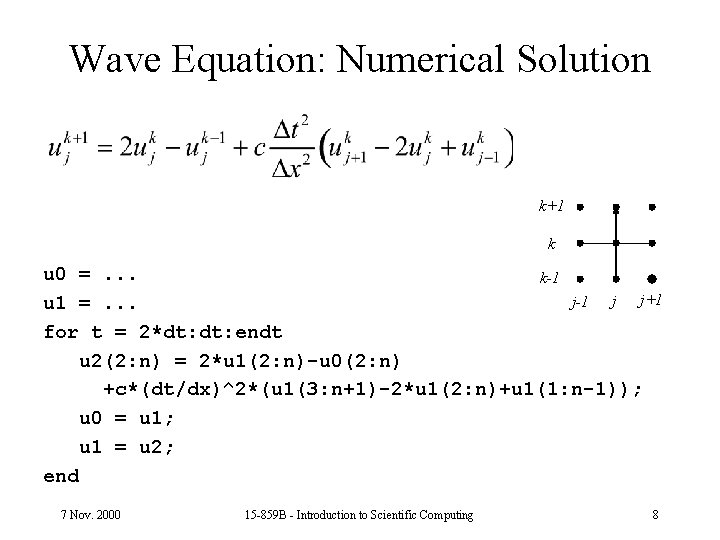Wave Equation: Numerical Solution k+1 k u 0 =. . . k-1 j j+1 u 1 =. . . for t = 2*dt: endt u 2(2: n) = 2*u 1(2: n)-u 0(2: n) +c*(dt/dx)^2*(u 1(3: n+1)-2*u 1(2: n)+u 1(1: n-1)); u 0 = u 1; u 1 = u 2; end 7 Nov. 2000 15 -859 B - Introduction to Scientific Computing 8Wave Equation Results dx=1/30 dt=. 01 7 Nov. 2000 15 -859 B - Introduction to Scientific Computing 9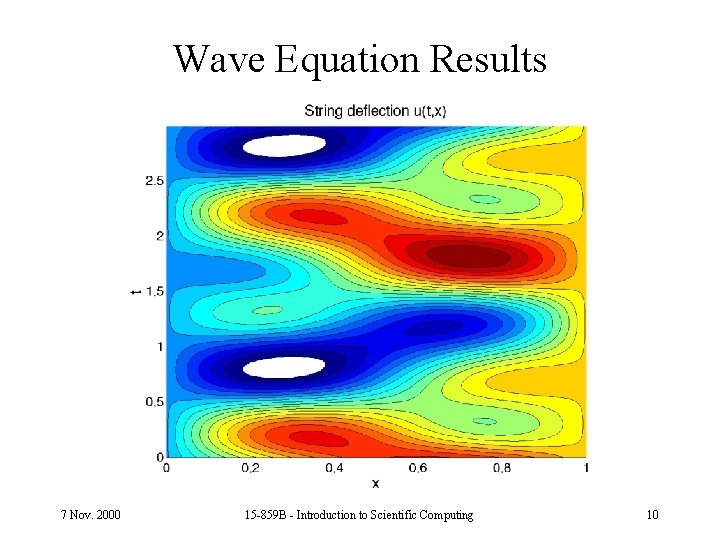Wave Equation Results 7 Nov. 2000 15 -859 B - Introduction to Scientific Computing 10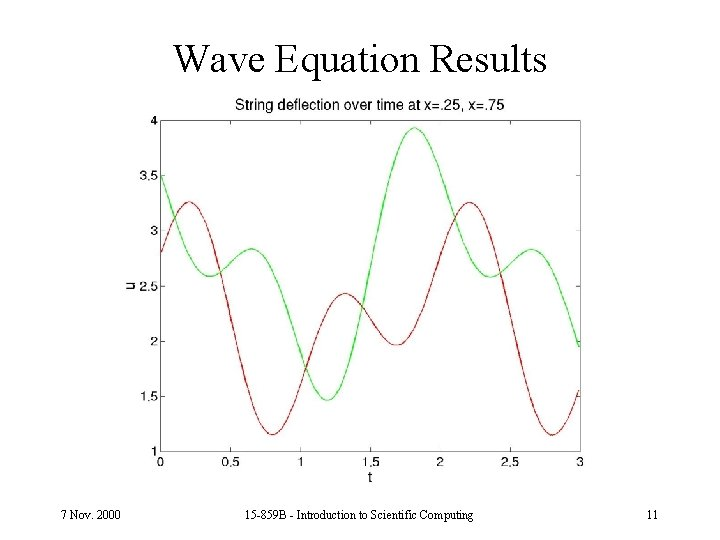Wave Equation Results 7 Nov. 2000 15 -859 B - Introduction to Scientific Computing 11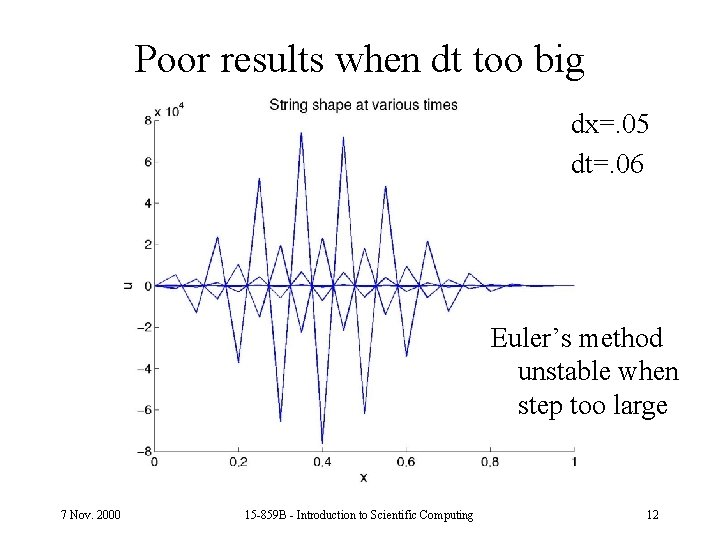Poor results when dt too big dx=. 05 dt=. 06 Euler’s method unstable when step too large 7 Nov. 2000 15 -859 B - Introduction to Scientific Computing 12PDE Solution Methods • Discretize in space, transform into system of IVP’s • Discretize in space and time, finite difference method. • Discretize in space and time, finite element method. – Latter methods yield sparse systems. • Sometimes the geometry and boundary conditions are simple (e. g. rectangular grid); • Sometimes they’re not (need mesh of triangles). 7 Nov. 2000 15 -859 B - Introduction to Scientific Computing 13